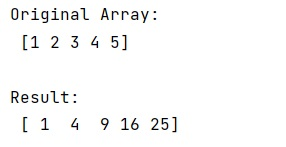# How to Map a Function Over NumPy Array?

In this tutorial, we will learn how to map a function over NumPy array? By Pranit Sharma Last updated : May 23, 2023

To map a function over NumPy array, the numpy.vectorize() method is used by passing a lambda expression in it.

## numpy.vectorize(): Map a Function Over NumPy Array

The following is the syntax of the function:

```class numpy.vectorize(pyfunc, otypes=None, doc=None, excluded=None, cache=False, signature=None)
```

Let us understand with the help of an example,

## Python Program to Map a Function Over NumPy Array

```# Import numpy
import numpy as np

# Creating a numpy array
arr = np.array([1, 2, 3, 4, 5])

# Display Original Array
print("Original Array:\n",arr,"\n")

# writing an expression
exp = lambda x: x ** 2

# Using numpy.vectorize
res = np.vectorize(exp)

# Call vectorized result
res = res(arr)

# Display Result
print('Result:\n',res)
```

### Output

```Original Array:
[1 2 3 4 5]

Result:
[ 1  4  9 16 25]
```

### Output (Screenshot)